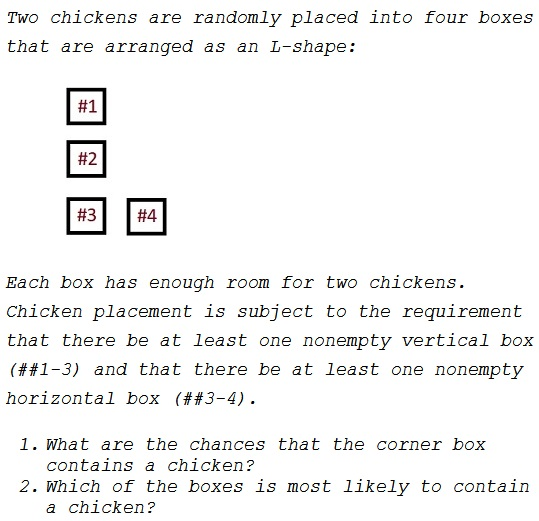# Two Chickens in Bigger Boxes

### Problem### Solution 1

As in the earlier problem, there are $16$ equiprobable chicken placements, of which five satisfy the conditions of the problem:

$\begin{array}{ccccc} \textit{Box #}&1&2&3&4\\ &\text{chicken}&&&\text{chicken}\\ &&\text{chicken}&&\text{chicken}\\ &&&\text{chicken}&\text{chicken}\\ &\text{chicken}&&\text{chicken}\\ &&\text{chicken}&\text{chicken}\\ &&&\text{2 chickens}\\ \end{array}$

Thus boxes #1-2 contain a chicken with the probability $\displaystyle \frac{1}{3};$ for boxes #3, the probability is $\displaystyle \frac{2}{3};$ for box #4, it's $\displaystyle \frac{3}{6}=\frac{1}{2}.$

### Solution 2

Let's follow where the chickens go. Each has equal probabilities of getting into any of the four boxes. Some configurations are OK, some violate the constraints of the problem.

$\begin{array}{ccccc} \textit{chick 1 in box / chick 2 in box}\\ &1&2&3&4\\ 1&X&X&OK!&OK\\ 2&X&X&OK!&OK\\ 3&OK!&OK!&OK!&OK!\\ 4&OK&OK&OK!&X\\ \end{array}$

There are $11$ legitimate equiprobable situations; in $7$ of them the corner box is not empty, in $6$ box #4 is not empty and in $4$ boxes #1-2 are each not empty. Thus the probabilities for the four boxes not to be empty come out as $\displaystyle \frac{4}{11},\frac{4}{11},\frac{7}{11},\frac{6}{11}.$

### Note

Why there are two solutions and which is correct, as the two can't be correct at the same time. The difference between the two is in that the first one treats the chickens as indistinguishable the second presumes that they are different: chicken #1 and chicken #2.

I do not believe that the problem is suggestive of the idea of two different chickens, although Solution 2 has been posted several times. I included it here to give a reason to remark on that topic.

### Acknowledgment

This is a modification of a problem from P. J. Nahin's Will You Be Alive 10 Years from Now? (Princeton University Press, 2014).

The two solutions above give different answers. Which is right?